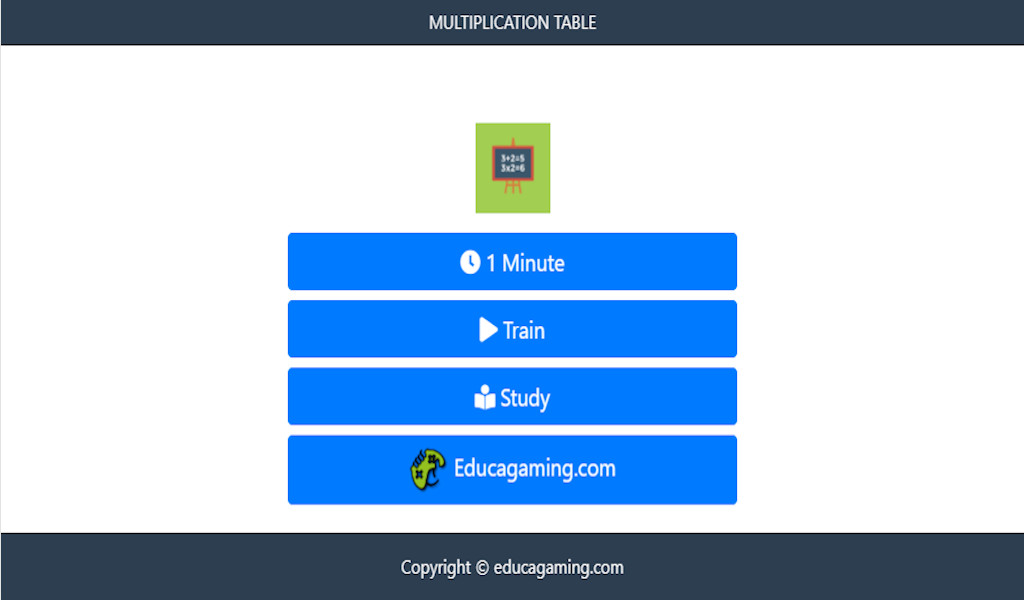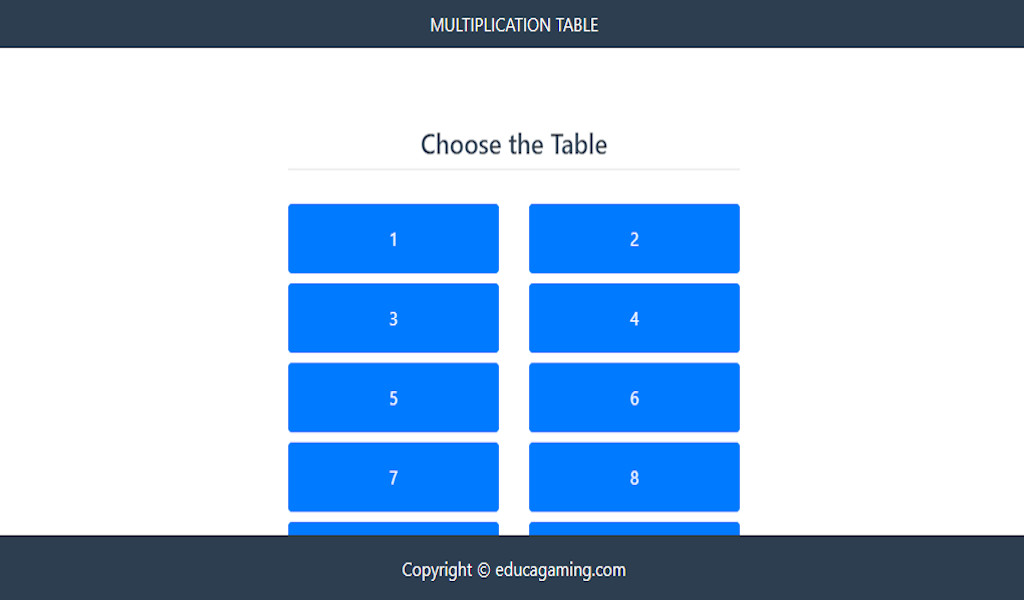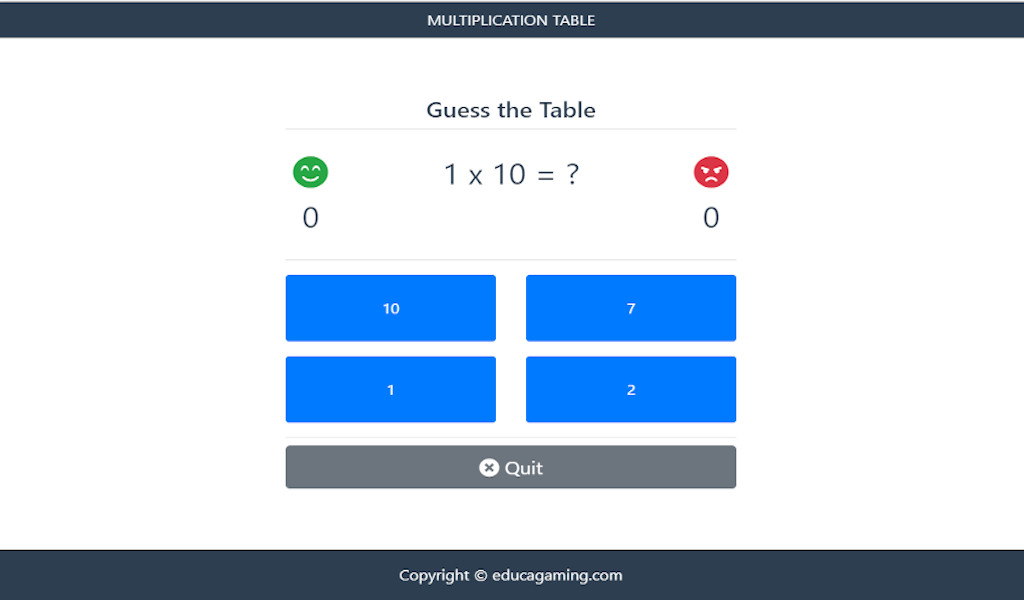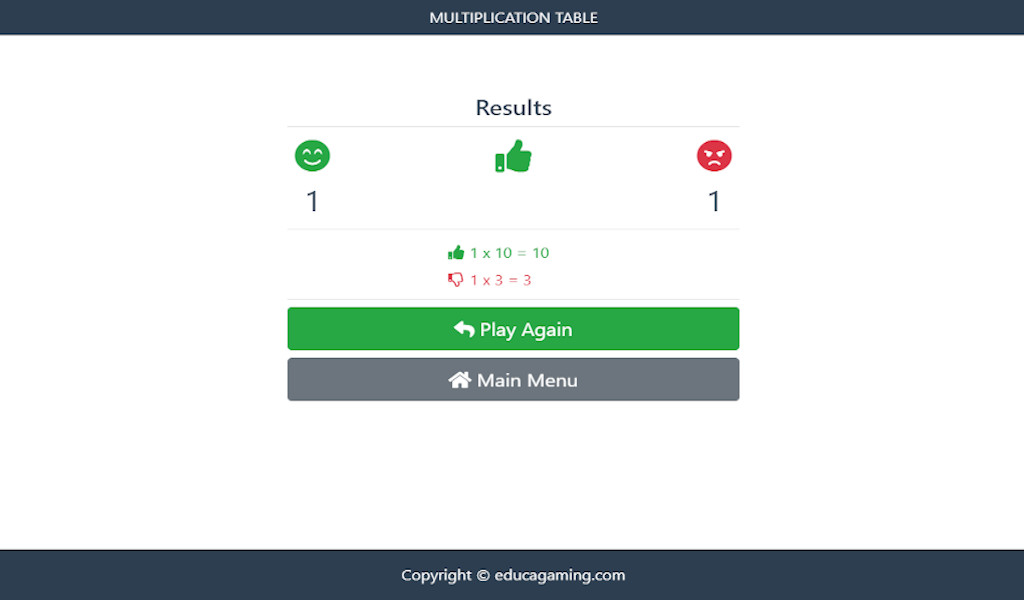## Math Tables Game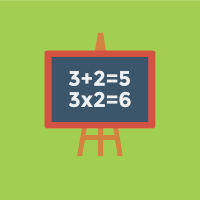##### Free Math Tables Educational Game

Math Tables Game is a educational game that helps us to decorate the times tables also brings us some fun facts about the times tables… let's go learn!

The times tables are a mathematical instrument based on a table used to define a multiplication operation in an algebraic system.

We know that there are ancient books that refer to the times tables as the times tables of Pythagoras.

Going back in time, the word tables originated in the tables of calculations used in Greece.

This table was created by Pythagoras, philosopher and mathematician, from the 6th century BC. C., at that time helping the commercial exchanges.

The concept has been following all times reaching the present day in a universal way for all of us.

Now that you've learned a little more about multiplication tables, let's have fun playing and learning this instrument that makes calculations easier!